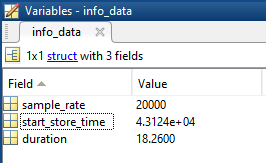A question that comes up very often by DWDataReader users is how can I read absolute time stamps. In this guide, we'll take a look at how we can do that in MATLAB, since that's a tool that's used very often among engineers performing data analysis.

As you've probably noticed, the time stamps that are returned by the default MATLAB script are relative, meaning that the first one starts at 0.00, while every next one increases by a factor of 1 divided by the sample rate (for synchronous channels). Apart from that, you can also read a struct called info_data, where you'll find a variable called start_store_time.On the picture above, you can see that the start_store_time variable contains a very large number. That value represents the absolute time in days since the 30th of December, 1899. The way that MATLAB interprets its absolute time is a bit different from the way it's done in Delphi, so we first need to convert this variable to a number that means something to MATLAB.We can do that by summing up the return of the datenum('30-Dec-1899') function and the info_data.start_store_time variable. This now becomes a number that MATLAB can interpret using the datestr() function, as can be seen in the image below.The date also matches up with the one displayed in DEWESoft. There is, however, an hour difference between the two, which can be explained by the local time display option, which I've selected inside DEWESoft's settings. As Slovenia lies in the UTC +1 time zone, this means that the local time will be one hour greater when compared to the regular UTC time format, which is stored inside the file.If you want to get each timestamp in the absolute format, you can convert it into the number of days, which is done by diving with a factor of (24*60^2) and then add each point to the absolute time offset.

From here, you can use any of the familiar MATLAB functions to perform date/time operations, such as datevec(), which will return a vector of the individual components.Finally, if you would like to store all of the time stamps in an absolute time format, you can add the absolute start variable to every element of the time stamp array. As the time stamps vector is stored as seconds by default, we first need to convert it into days. The image below shows how to do that with a single sample.That concludes this tutorial. If you have any further questions that you need clarification on, please write us to support@dewesoft.com, or submit a ticket through the support portal.